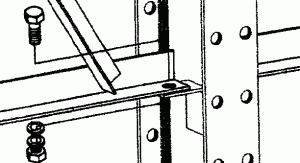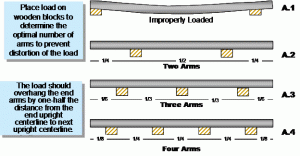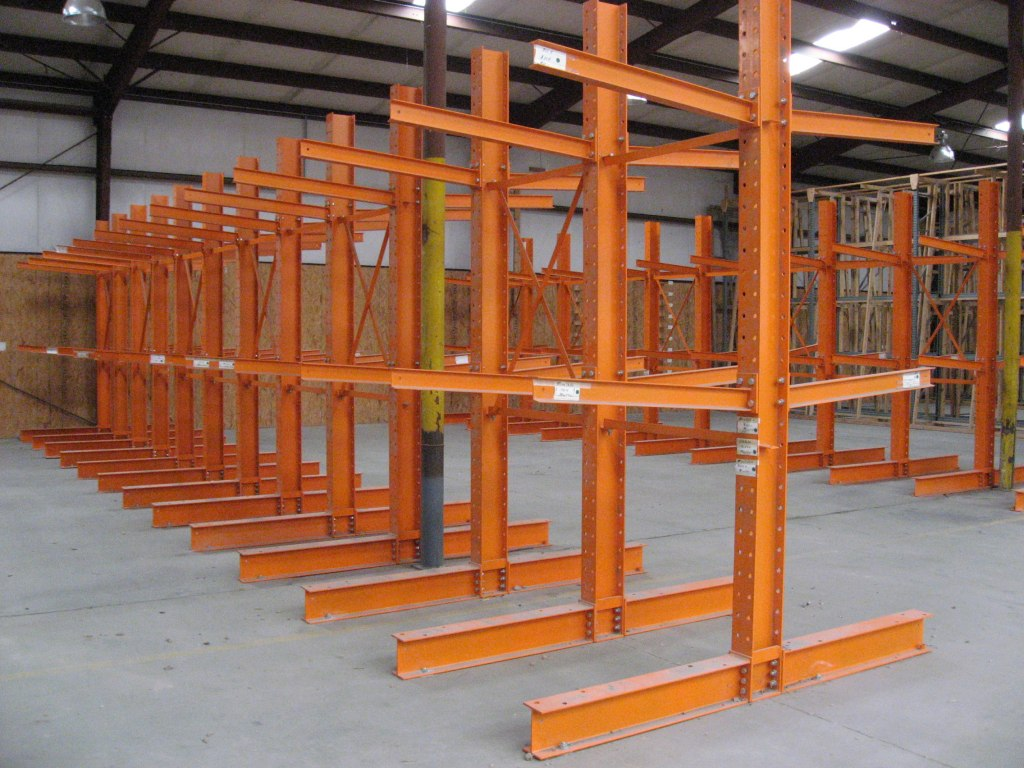The cantilever capacity guide will assist you in evenly distributing your load, determining cantilever arm and column capacities, ascertaining column load distribution, and establishing a maximum distance between columns.One Section of Single Sided Cantilever Rack
with 4 Columns and 3 Arm Levels

Loads must be evenly distributed in order to attain the capacities listed.
An uneven load reduces the capacity by 50%.Floor Anchors must be used in all base and column “anchor holes” to attain capacities.
Type of floor anchors required is determined by slab construction and therefore must be determined by customer’s engineer.

B. Arm Capacities
Cantilever Arm Capacity
Cantilever Arm Capacity Chart48″x4″ cantilever arm 2,000 pounds per arm

Each cantilever arm can support 2,000 pounds*.
In diagram 1, if the load spanned the entire horizontal length of the cantilever section, the arms could support a load weighing up to 8,000 pounds. (load supported by 4 arms x 2,000 pounds per arm = 8,000 pounds)

It is necessary to consider column capacities as well as arm capacities in order to prevent overloading cantilever rack.

C. Column Capacities
Cantilever Column Capacity
Cantilever Column Capacity Chart
12′ tall Cantilever Column 10,200 Pounds per side (see load distribution info)
16′ tall Cantilever Column 8,800 Pounds per side (see load distribution info)

Determine how much weight each column is actually supporting.
Load Distribution per column must be calculated in order to determine how much weight is being supported by each individual cantilever column.Determine the weight being stored on each of the 3 columns.
Step 1. Divide the total load weight by the number of columns supporting the load.
Step 2. Add the weight of any loads which are only supported by one column. (yellow loads)

Example:
Column #1 is supporting 1/2 of load a plus 400 pounds:
Load a = 5000 pounds divided by 2 columns = 2,500 pounds
column #1 = 400 pounds
Column #1 is supporting 2,500 pounds + 400 pounds = 2,900 pounds

Column #2 is supporting 1/2 of load a plus 1/2 of load b
plus 400 pounds + 200 pounds
Load a = 5000 pounds divided by 2 columns = 2,500 pounds
Load b = 3,000 pounds divided by 2 columns = 1,500 pounds
column #2 : = 400 pounds + 200 pounds = 600 pounds
Column #2 is supporting 2,500 pounds + 1,500 pounds + 600 pounds = 4,600 pounds

Column #3 is supporting 1/2 of load b plus 200 pounds
Load b = 3,000 pounds divided by 2 columns = 1,500 pounds
column #3 = 200 pounds
Column #3 is supporting 1,500 pounds + 200 pounds = 1,700 pounds

Total Weight Stored on Each Column in Example
Column #1 Column #2 Column #3
2,900# 4,600# 1,700#
Neither of the 3 columns support more than 10,200 pounds per side, therefore the cantilever in diagram #3 is loaded within the capacity limits.

CANTILEVER COLUMN CAPACITIES ARE STATED PER SIDE.
(see diagram 4)
Example: A 12′ tall Middle Cantilever Column has a capacity of 10,200 pounds if the column is single sided, and a capacity of 10,200 pounds PER SIDE if the column is double sided.
Single Sided Double SidedLoads which are stored on the base (not on the arm) are not
supported by the column. Therefore, “base loads” should not
be considered when determining column capacity.

E. Determining Maximum Distance Between Columns
Distance between columns (bracing size, see diagram 4) should not exceed a dimension equal to:

Length of the load divided by
Total # of Columns

In Diagram 4, the distance between the columns must not exceed:

15 feet (length of the load)
2 columns (# of columns supporting the load)

Distance Between Columns may not exceed 7 1/2 feet.